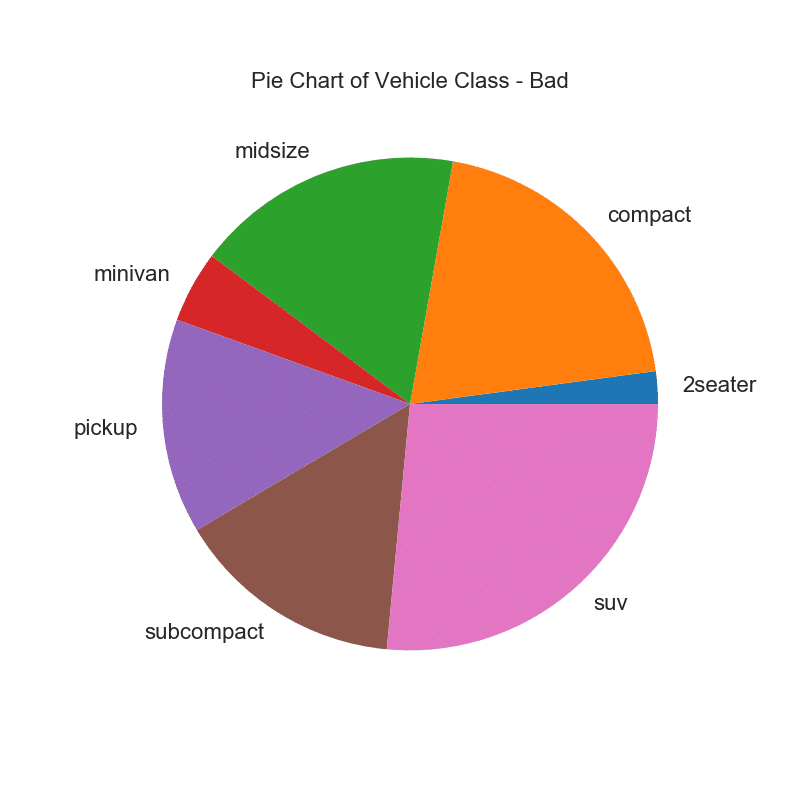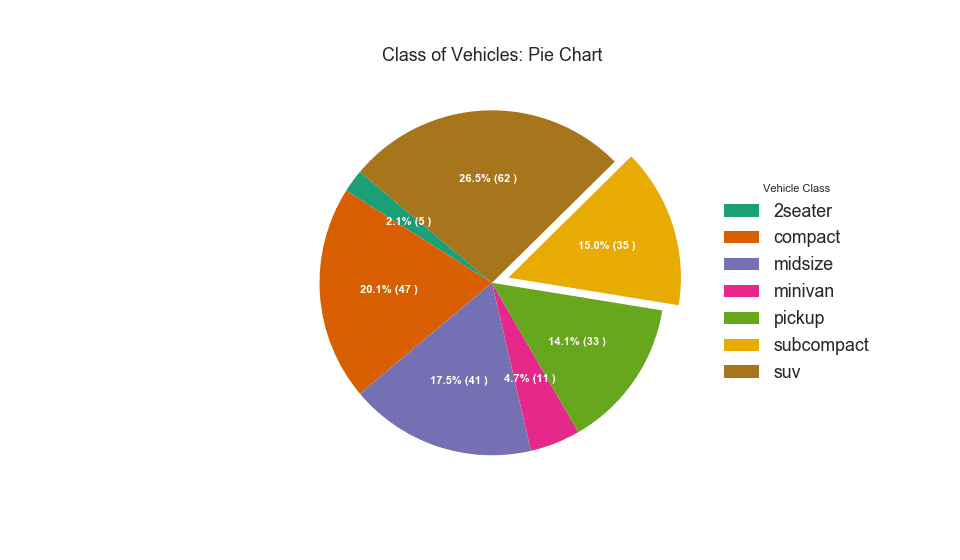Hits: 95

# Python Data Visualisation for Business Analyst – How to do Pie Plot in Python

In this data visualisation tutorial, How to do Pie Plot in Python.

Pie chart is a classic way to show the composition of groups. However, its not generally advisable to use nowadays because the area of the pie portions can sometimes become misleading. So, if you are to use pie chart, its highly recommended to explicitly write down the percentage or numbers for each portion of the pie.

## Setup

Run this once before the plot’s code. The individual charts, however, may redefine its own aesthetics.

``````/* !pip install brewer2mpl */
import numpy as np
import pandas as pd
import matplotlib as mpl
import matplotlib.pyplot as plt
import seaborn as sns
import warnings; warnings.filterwarnings(action='once')

large = 22; med = 16; small = 12
params = {'axes.titlesize': large,
'legend.fontsize': med,
'figure.figsize': (16, 10),
'axes.labelsize': med,
'axes.titlesize': med,
'xtick.labelsize': med,
'ytick.labelsize': med,
'figure.titlesize': large}
plt.rcParams.update(params)
plt.style.use('seaborn-whitegrid')
sns.set_style("white")
%matplotlib inline

/* Version */
print(mpl.__version__)
print(sns.__version__)``````

## How to do Pie Plot in Python

``````/* Import */

/* Prepare Data */
df = df_raw.groupby('class').size()

/* Make the plot with pandas */
df.plot(kind='pie', subplots=True, figsize=(8, 8), dpi= 80)
plt.title("Pie Chart of Vehicle Class - Bad")
plt.ylabel("")
plt.show()``````Show Code

``````/* Import */

/* Prepare Data */
df = df_raw.groupby('class').size().reset_index(name='counts')

/* Draw Plot */
fig, ax = plt.subplots(figsize=(12, 7), subplot_kw=dict(aspect="equal"), dpi= 80)

data = df['counts']
categories = df['class']
explode = [0,0,0,0,0,0.1,0]

def func(pct, allvals):
absolute = int(pct/100.*np.sum(allvals))
return "{:.1f}% ({:d} )".format(pct, absolute)

wedges, texts, autotexts = ax.pie(data,
autopct=lambda pct: func(pct, data),
textprops=dict(color="w"),
colors=plt.cm.Dark2.colors,
startangle=140,
explode=explode)

/* Decoration */
ax.legend(wedges, categories, title="Vehicle Class", loc="center left", bbox_to_anchor=(1, 0, 0.5, 1))
plt.setp(autotexts, size=10, weight=700)
ax.set_title("Class of Vehicles: Pie Chart")
plt.show()``````# Personal Career & Learning Guide for Data Analyst, Data Engineer and Data Scientist

## Applied Machine Learning & Data Science Projects and Coding Recipes for Beginners

A list of FREE programming examples together with eTutorials & eBooks @ SETScholars

# Projects and Coding Recipes, eTutorials and eBooks: The best All-in-One resources for Data Analyst, Data Scientist, Machine Learning Engineer and Software Developer

Topics included: Classification, Clustering, Regression, Forecasting, Algorithms, Data Structures, Data Analytics & Data Science, Deep Learning, Machine Learning, Programming Languages and Software Tools & Packages.
(Discount is valid for limited time only)`Disclaimer: The information and code presented within this recipe/tutorial is only for educational and coaching purposes for beginners and developers. Anyone can practice and apply the recipe/tutorial presented here, but the reader is taking full responsibility for his/her actions. The author (content curator) of this recipe (code / program) has made every effort to ensure the accuracy of the information was correct at time of publication. The author (content curator) does not assume and hereby disclaims any liability to any party for any loss, damage, or disruption caused by errors or omissions, whether such errors or omissions result from accident, negligence, or any other cause. The information presented here could also be found in public knowledge domains.`

# Learn by Coding: v-Tutorials on Applied Machine Learning and Data Science for Beginners

Please do not waste your valuable time by watching videos, rather use end-to-end (Python and R) recipes from Professional Data Scientists to practice coding, and land the most demandable jobs in the fields of Predictive analytics & AI (Machine Learning and Data Science).

The objective is to guide the developers & analysts to “Learn how to Code” for Applied AI using end-to-end coding solutions, and unlock the world of opportunities!

How to create PIE chart in R

R Visualisation for Beginners – Pie Charts

Beginners Guide to R – R Pie Chart – Base Graph